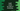# How to create an array of dictionary elements in swiftArrays are used to hold elements of a single type. We can have an array of strings, characters, integers etc. In Swift, we can easily create one array using array literal i.e. put the elements of the array inside a square bracket.

Again, dictionary in Swift is a collection that holds key-value pairs. Using a key, we can read or modify the value associated with it. Swift provides an easy way to create a dictionary by putting the key-value pairs inside a square bracket. One colon is used to separate each key from its associated value.

If we put dictionaries in an array, that will make it array of dictionaries . In this post, I will show you how to create an array of dictionary with example.

## Array of dictionary :

Nested square bracket is used to create an array of dictionary. Let’s take a look at the below example :

``````var dict_one: [String: Int] = ["one": 1, "two": 2, "three": 3]
var dict_two: [String: Int] = ["four": 4, "five": 5, "six": 6]

var dict_arr: [[String: Int]] = [dict_one, dict_two]

if let one = dict_arr["one"],let six = dict_arr["six"]{
print(one)
print(six)
}``````

### Explanation :

• We have two dictionaries of type String: Int with different values.
• dict_arr is an array that can hold dictionary elements of type String: Int. Using array literal, we initialized it with dict_one and dict_two.
• Dictionary returns optional value. So, we are safe unwrapping it before printing the content.

It will print the below output :

``````1
6``````